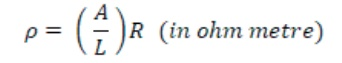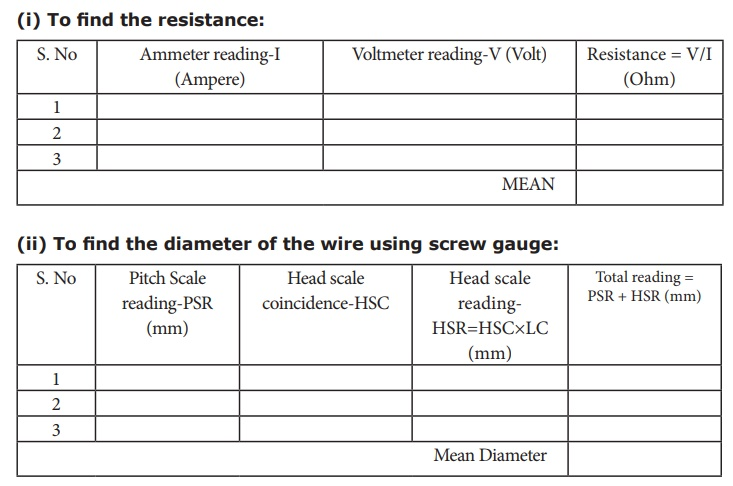Home | | Science 10th Std | Determination of resistivity

# Determination of resistivity

To determine the resistivity of the material of the given coil of wire.

DETERMINATION OF RESISTIVITY

### Objective:

To determine the resistivity of the material of the given coil of wire.

### Equipment required:

A coil of wire, screw gauge, a metre scale, battery, key, ammeter, voltmeter, rheostat and connecting Wires.

### Formula:

The resistivity of the material of the coil of wire isWhere A is the area of cross section of the wire (m2)

L is the length of the coil of wire (m)

R is the resistance of the coil of wire (ohm)

### Procedure:

·                 Connect the battery, ammeter, given wire, rheostat and key in series, as shown in the circuit diagram.

·                 Connect the voltmeter in parallel to the unknown resistor.·                 Close the key and hence the circuit is closed.

·                 Adjust the rheostat such that the ammeter reads a current of 0.5 ampere.

·                 Note down the potential difference across the resistor as shown by the voltmeter.

·                 Adjust the rheostat and change the current in steps of 0.5A (that is 0.5A, 1.0A, 1.5A, etc.).

·                 For each current, note down the corresponding potential difference as shown by the voltmeter.

·                 Tabulate the observations.

·                 Measure the diameter of the wire using a screw gauge.

·                 Measure the length of the coil using metre scale

### (i) To find the resistance: (ii) To find the diameter of the wire using screw gauge:### Calculations:

Radius of the wire, r = diameter/2 = _____________ m

Area of cross section of the wire, A = π r2 = ____________ m2

Length of the wire L = __________ m.

Resistivity of the material of the wire == __________ Ω m

### Result:

The resistivity of the material of the wire = __________ Ω m

Tags : Physics Laboratory Practical Experiment , 10th Science : Physics Practicals
Study Material, Lecturing Notes, Assignment, Reference, Wiki description explanation, brief detail
10th Science : Physics Practicals : Determination of resistivity | Physics Laboratory Practical Experiment